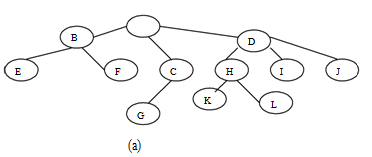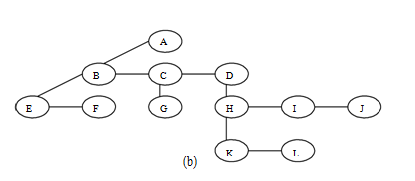## Conversion of general trees into the binary trees, Data Structure & Algorithms

Assignment Help:

By taking an appropriate example explain how a general tree can be represented as a Binary Tree.

Conversion of general trees into the binary trees:

A general tree can be changed into an equivalent binary tree. This conversion process or technique is called the natural correspondence between general and binary trees.

The algorithm is written below:

(a) Insert the edges connecting siblings from left to right at the same level.

(b Erase all edges of a parent to its children except to its left most offspring.

(c) Rotate the obtained tree 450 to mark clearly left and right subtrees.

A general tree shown in figure (a) converted into the binary tree shown in (b)#### Cohen sutherland algorithm, Using the cohen sutherland. Algorithm. Find the...

Using the cohen sutherland. Algorithm. Find the visible portion of the line P(40,80) Q(120,30) inside the window is defined as ABCD A(20,20),B(60,20),C(60,40)and D(20,40)

#### Avl tree, Example: (Single rotation into AVL tree, while a new node is inse...

Example: (Single rotation into AVL tree, while a new node is inserted into the AVL tree (LL Rotation)) Figure: LL Rotation The rectangles marked A, B & C are trees

#### Nested for loop, nested for loop for (i = 0; i for (j = 0; j seq...

nested for loop for (i = 0; i for (j = 0; j sequence of statements } } Here, we observe that, the outer loop executes n times. Every time the outer loop execute

#### Linked list implementation of a dequeue, Double ended queues are implemente...

Double ended queues are implemented along doubly linked lists. A doubly link list can traverse in both of the directions as it contain two pointers namely left pointers and righ

#### frequenty count of function, Ask question find frequency count of function...

Ask question find frequency count of function- {for(i=1;i {for(j=1;j {for(k=1;k } } }

#### How do you rotate a binary tree, How do you rotate a Binary Tree?  Rot...

How do you rotate a Binary Tree?  Rotations in the tree: If after inserting a node in a Binary search tree, the balancing factor (height of left subtree - height of right

#### Explain all-pair shortest-paths problem, Explain All-pair shortest-paths pr...

Explain All-pair shortest-paths problem Given a weighted linked graph (undirected or directed), the all pairs shortest paths problem asks to find the distances (the lengths of

#### Explain np-complete decision problem, a. Determine the result of inserting ...

a. Determine the result of inserting the keys 4,19, 17, 11, 3, 12, 8, 20, 22, 23, 13, 18, 14, 16, 1, 2, 24, 25, 26, 5 in order to an empty B-Tree of degree 3. Only draw the configu

#### Binary tree traversals, We have discussed already about three tree traversa...

We have discussed already about three tree traversal methods in the earlier section on general tree. The similar three different ways to do the traversal -inorder , preorder, and p

#### Multiple stacks, how multiple stacks can be implemented using one dimension...

how multiple stacks can be implemented using one dimensional array